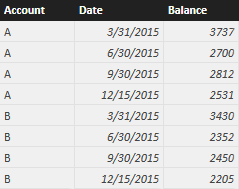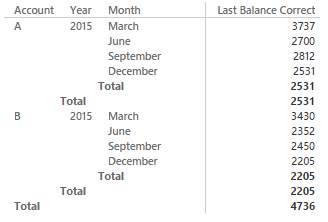The LASTDATE function in DAX retrieves the last date in the filter context. In this puzzle, you have to fix a wrong measure where LASTDATE is not working as expected.

## Scenario

The data model has a simple Accounts table with 8 rows (one for the last available date in each quarter) and 3 columns: Account, Date, and Balance.The data model does not contain a Date table, even if an additional Month column is automatically created by Excel 2016 and Power BI Desktop.

## The Challenge

The data model has a measure that should display the sum of the last available date in the period selected in a report. This is the definition of such a measure:

```Last Balance :=
CALCULATE (
SUM ( Accounts[Balance] ),
FILTER ( ALL ( Accounts ), Accounts[Date] = LASTDATE ( Accounts[Date] ) ),
VALUES ( Accounts[Account] )
)
```

However, using this measure the result of the following report is wrong.As you see, the Last Balance measure display a wrong result, which is the sum of all the dates for each account, regardless of the filter over date.
The expected result is the following one, where you see that the totals at the year level and at the account level correspond to the month of December:You have to fix the formula by only replacing one function name, without modifying the structure of the measure.

## Hints

You can obtain the right result in many different ways. It is ok to try different approaches, but before looking at the solution, try to understand the exact reason why the current measure does not work, and what is the single change you have to apply to make this formula working, without altering its original design.

The zip file for this puzzle contains examples in both Power BI and Excel.

## Solution

To see the solution, just press the button below.